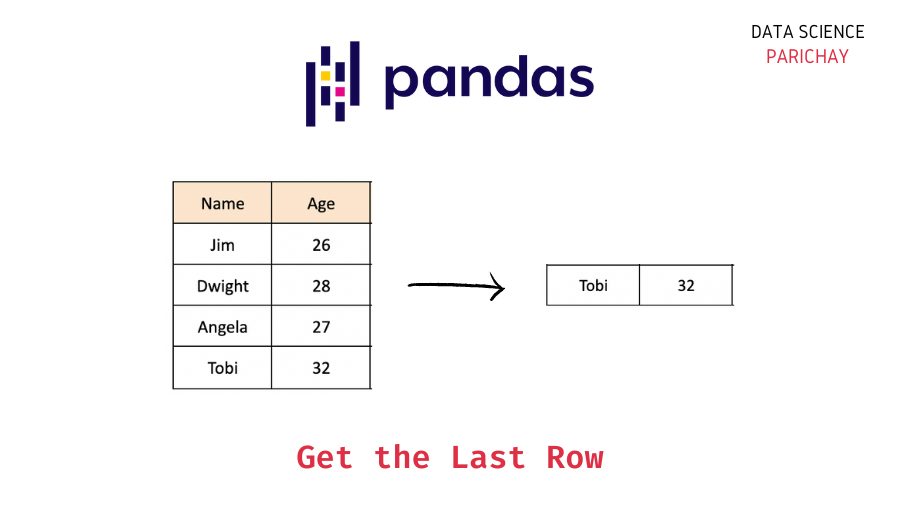# Pandas – Get the Last Row of a Dataframe

Selecting rows from a dataframe is now one of the most common tasks anyone can do with pandas. In this tutorial, we will look at how to get the last row of a dataframe in pandas with the help of some examples.

## Select the last row of a dataframe

Sometimes you may need to select the last row of a pandas data frame. There are multiple ways to select the last row of a dataframe. For example –

• Use the pandas dataframe `iloc` property.
• Use the pandas `tail()` function.
```# Using the iloc[] Property to Retrieve the DataFrame’s Last Row
df.iloc[-1]

# Retrieve the Last Row of All or a Specific Column Using the tail() Function
df.tail(1)```

## Examples

Let’s now look at some examples of using the above syntax.

First, we will create a pandas dataframe that we will be using throughout this tutorial.

```import pandas as pd

# employee data
data = {
"Name": ["Ram", "Sita", "Anita", "Abhinash"],
"Age": [26, 28, 27, 32],
"Department": ["Sales", "Sales", "Accounting", "HR"]
}

# create pandas dataframe
df = pd.DataFrame(data)

# display the dataframe
df```

Output:

Here, we created a dataframe with data with information on some employees in an office. You can see that the column names in the above dataframe are – “Name”, “Age”, and “Department”.

### Example 1: Get the Last Row of a Dataframe using the `iloc[]` property

The Pandas module in Python defines the `iloc[]` property which allows you to retrieve a specific column or row from the given DataFrame. Using the index values, we can quickly extract any specific value from a column or a row using the `iloc[]` property.

The following code shows how to get the last row of a dataframe using the `iloc[]` property.

📚 Data Science Programs By Skill Level

Introductory

Intermediate ⭐⭐⭐

🔎 Find Data Science Programs 👨‍💻 111,889 already enrolled

Disclaimer: Data Science Parichay is reader supported. When you purchase a course through a link on this site, we may earn a small commission at no additional cost to you. Earned commissions help support this website and its team of writers.

```# Using the iloc[] to Retrieve the DataFrame’s Last Row
df.iloc[-1]```

Output:

```Name          Abhinash
Age                 32
Department          HR
Name: 3, dtype: object```

Pandas dataframe rows are indexed starting from 0. Thus, the index of the first row is 0 and that of the last row is n-1 where n is the length of the dataframe. Using a negative index can be helpful here. The index -1 represents the index of the first row from the end of the dataframe, that is, the last row of the dataframe.

So, we can use -1 as the index inside the `iloc[]` property to get the last row of the “df” DataFrame.

You can similarly get the last value (value in the last row) of a specific column in a pandas dataframe using the `iloc[]` property.

```# Retrieve the Last Row of Name Column Using the iloc[] property
df['Name'].iloc[-1]```

Output:

`'Abhinash'`

### Example 2: Get the Last Row of a Dataframe using the `tail()` function

The `tail()` function in pandas retrieves the last “n” rows of a dataframe. The last “n” (the default value is 5) DataFrame’s rows or series are returned using this method. To retrieve just the last row, we pass 1 as an argument to the `tail()` function. The following code shows the same.

```# Retrieve the Last Row Using the tail() Function
df.tail(1)```

Output:

By using the value of 1 inside the `tail()` function, we retrieved the last row. Just like with the `iloc[]` property, we can also use the `tail()` function to retrieve the last row of a specific column of the DataFrame.

```# Retrieve the Last Row of Name Column Using the tail() Function
df['Name'].tail(1)```

Output:

```3    Abhinash
Name: Name, dtype: object```

We get the last value in the “Name” column of the above dataframe.

## Summary

In this tutorial, we looked at how to access the last row of a pandas dataframe using the following methods –

• Using the `iloc[]` property.
• Using the `tail()` function.

We also looked at how to get the value in the last row for a specific column using the above methods.

You might also be interested in –

•